# Feature Scaling with Scikit-Learn for Data Science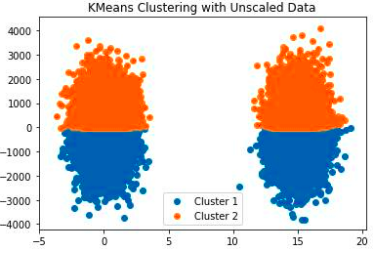Do you really want to this happen?

In the data science process, we need to do some preprocessing before machine learning algorithms. These can be some basic data analysis processes such as handling missing values and outliers and data cleaning. We also apply scaling (data transformation) for some data.

Scaling is not mandatory, but it performs better to scale the data before some machine learning algorithms.

The main purpose of scaling is to avoid the effects of greater numeric ranges. Especially it is so important to machine learning algorithms which the distance is important, such as KNN (k Nearest Neighbor), K-Means Clustering, SVM (Support Vector Machine), PCA (Principal Component Analysis) algorithms.

In the scatter plots below, you can check the difference between scaling and non-scaling. Scaling was used in the PCA process. The range(scale) is very wide without scaling so it is difficult to separate points. When scaling is used, it provides efficiency and good performance.

As stated before, the purpose of scaling is to bring each data in the dataset closer together. The other goal is to avoid some types of numerical difficulties during the calculation. For example, Gradient Descent based optimization algorithm likes to scale and performs better with scaling. Linear Regression and Logistic Regression, common algorithms using Gradient Descent, can be scaled too. We can also apply scaling for other machine learning algorithms and Neural Networks. We can achieve better performance and better results. We can compare results with scaling and without scaling.

Some algorithms like Decision Tree, Random Forest, Adaboost do not need to scale.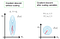Reference

We will focus on the most common; StandardScaler, RobustScaler, MinMaxScaler, and MaxAbsScaler.

1 — StandardScaler

`from sklearn.preprocessing import StandardScaler`

StandardScaler is a mean-based scaling method. The formula of StandardScaler is (Xi-Xmean)/Xstd, so it adjusts the mean as a 0.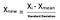Reference

The StandardScaler is vulnerable to outliers because outliers affect the mean. If you have a normal distribution or have near-normal data, StandardScaler moves your data closer to the standard normal distribution. However, StandardScaler will not perform well if you have outliers.

Let’s check the StandardScaler on charts;

`from sklearn.preprocessing import StandardScalerdf_scale = StandardScaler().fit_transform(df)`

We have right-skewed data. After StandardScaler implementation our data are clustered around 0, but it is still right-skewed and has a large scale (-1 and 30). The data are clustered and do not have large outliers. If we have larger values as outliers, we cannot get good results with StandardScaler. Because outliers will affect all scaling data. It also affected this data. In such cases, it is better to remove outliers firstly. For now, we will use log transformation. You can check this link for the methods of dealing with outliers.

`from sklearn.preprocessing import StandardScalerimport numpy as npdf_log = np.log(df)df_scale = StandardScaler().fit_transform(df_log)`

The log transformation changes data distribution to the normal distribution. After the log transformation, the StandardScaler implementation makes our data standard normal distribution (near standard normal distribution). This method performs better and removes the effects of outliers. It is better to apply StandardScaler to data that are not outliers.

2 — RobustScaler

`from sklearn.preprocessing import RobustScaler`

This Scaler removes the median and scales the data according to the quantile range (defaults to IQR: Interquartile Range). The IQR is the range between the 1st quartile (25th quantile) and the 3rd quartile (75th quantile).

RobustScaler is a median-based scaling method. The formula of RobustScaler is (Xi-Xmedian) / Xiqr, so it is not affected by outliers.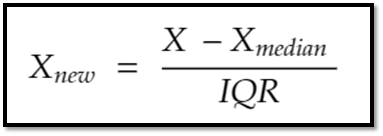Reference

Since it uses the interquartile range, it absorbs the effects of outliers while scaling. The interquartile range (Q3 — Q1) has half the data point. If you have outliers that might affect your results or statistics and don’t want to remove them, RobustScaler is the best choice.

Above, the original data (without scale) have outliers at points 250000 and 200000, after RobustScaler they change to 150 and 200, but StandardScaler makes them 25 and 35. Outliers affect the StandardScaler and get too close to the mean point. RobustScaler keeps outliers where they should be away from the data mean.

3 — MinMaxScaler

`from sklearn.preprocessing import MinMaxScaler`

This estimator scales and translates each feature individually such that it is in the given range on the training set, e.g. between zero and one.

The formula of MinMaxScaler is (Xi-Xmin) / (Xmax-Xmin).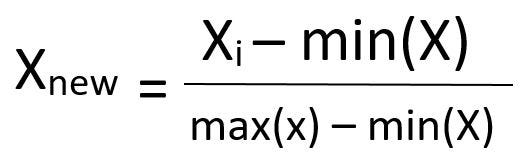Reference

MinMaxScaler sets data from 0 to 1. Scaling is calculated with minimum and maximum points, therefore data can be affected by outliers. It is not suitable for outliers. Before implementing MinMaxScaler, it is good to handle outliers.

4— MaxAbsScaler

`from sklearn.preprocessing import MaxAbsScaler`

The formula of MaxAbsScaler is Xi /|Xmax|. If the data has negative values, MaxAbsScaler sets the data between -1 and 1. It scales data according to the absolute maximum, so it is not suitable for outliers. It needs pre-processing like handling with outliers.

Conclusion

The principal purpose of scaling is to restate each variable on a standardized scale and to avoid numerical instabilities potentially caused by too large numbers, to speed up optimization algorithms. And the most significant issue about scaling is that be sure that apply scaling both of training and test data. We want to scale all data to the same scale.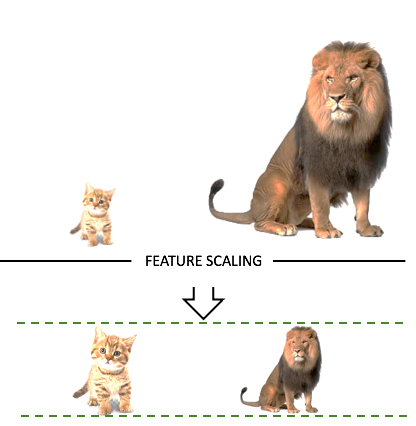Reference

StandardScaler

• It is not suitable for data that have outliers.
• It adjusts the mean to 0.

RobustScaler

• It is more suitable for data that have outliers.

MinMaxScaler

• It is not suitable for data that have outliers.
• It adjusts the data between 0 and 1.

MaxAbsScaler

• It is not suitable for data that have outliers.
• It adjusts the data between -1 and 1.

Data Scientist | Machine Learning Proficiency | Industrial Engineer

## More from Hasan Ersan YAĞCI

Data Scientist | Machine Learning Proficiency | Industrial Engineer